Chemguide: Support for CIE A level Chemistry ``` ``` Learning outcome 28: Chemistry of transition elements 28.2: General characteristic chemical properties of the first set of transition elements, titanium to copper ``` ``` Learning outcomes 28.2.9 and 28.2.10 Statement 28.2.9 lists three examples of redox reactions involving transition element compounds. They expect you to be able to describe the reactions and carry out calculations on them. Statement 28.2.10 extends this to possibly unfamiliar reactions where you are given the necessary information in the question. ``` ``` Important background: Writing equations for redox reactions This statement will expect you to work out the equations for redox reactions using half-equations. If you aren't confident about this, then you must read the page about writing ionic equations for redox reactions before you go on. For CIE purposes, you probably won't have to work out the half-equations for yourself. For inorganic reactions, you will probably either be given the half-equation, or you can find it from the Data Booklet which you will have in the exam. You will find a copy of this booklet towards the end of the syllabus. For organic reactions, you probably won't be asked to write these ionic equations anyway. The teacher support material says that oxidation of, for example, alcohols can be written in terms of [O] instead of a proper equation. Example 1 One of the examples that you might have just read about on the page about writing ionic equations was the reaction between acidified potassium manganate(VII) and hydrogen peroxide. Oxygen is produced, and the manganate(VII) ions are reduced to manganese(II) ions. In the example on that page, you had to work out the half-equations for yourself. If you have the Data Booklet, how do you go about finding them? First, find the copy of the Data Booklet towards the end of the syllabus, and find the table headed "Standard electrode potential and redox potentials" Then you need to think about the redox reaction in two halves. You need to find an equation which involves MnO4- ions and Mn2+ ions (and hydrogen ions as well, because it is done under acidic conditions). All the reactions in this table are shown as equilibria. That means that you could make them move in either direction. So it doesn't matter which side of the equation the MnO4- and Mn2+ appear on - as long as they are on opposite sides. Look through the table (bearing in mind that it is in alphabetical order), and you should find: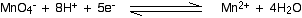That is actually the change you want to happen, so you can replace the reversible sign by a one-way arrow.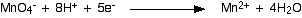Now do the same thing for the hydrogen peroxide reaction. You need an equation with hydrogen peroxide on one side and oxygen on the other. You should find: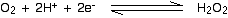That's the reverse of what you want to happen, so turn it around and replace the reversible sign with a one-way arrow.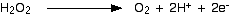Now you can combine these equations together as you were shown on the page about working out ionic equations for redox reactions. This is good practice. Do it now and then look at that page again to see if you got it right. If you didn't, spend some more time on that page before you go any further. Example 2 This comes from a past paper and was worth 2 marks. You were told that copper reacts with sodium vanadate(V) solution, NaVO3, to give a solution containing VO2+ ions and Cu2+ ions, and had to work out the equation. Because of the formula of the sodium vanadate(V), the vanadate(V) ions must carry a charge of -1. So the first thing you have to do is to find a half-equation for the change from VO3- to VO2+. That's not difficult. You should find: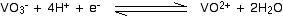That is the direction you want it to go in, so you can change it to: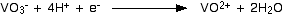You could reasonably make up the copper half-equation for yourself.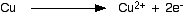Or you could find it in the table. You would have to remember to reverse the direction, though. Now all you have to do is to add them together, remembering that you need to make sure that the number of electrons being given and received by the two equations is the same. The vanadate(V) reaction would have to happen twice to make this work.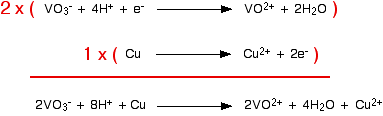``` ``` Statement 28.2.9(a) This reaction is used to standardise (accurately find the concentration of) potassium manganate(VII) solution to be used in titrations. Potassium manganate(VII) isn't a primary standard. That means that it can't be made up to give a stable solution of accurately known concentration. It is so strongly coloured that it is impossible to see when all the crystals you have used have dissolved, and over a period of time it oxidises the water it is dissolved in to oxygen. Bottles of potassium manganate(VII) solution usually have a brown precipitate around the top. This is manganese(IV) oxide - and is produced when the manganate(VII) ions react with the water. You have to make up a solution which is approximately what you want, and then standardise it by doing a titration. This is often done with a solution of sodium ethanedioate, because this is a primary standard. Practical details The potassium manganate(VII) solution is placed in the burette, and sodium ethanedioate solution of accurately known concentration is pipetted into a flask. The solution in the flask is acidified with some dilute sulfuric acid. The quantity isn't critical, but it has to be an excess. The reaction has to be done hot, and so the solution in the flask is heated to about 60°C - that is hot enough so that if you hold the flask in the palm of our hand, it hurts after about a second or two! The purple potassium manganate(VII) solution is run in to the flask where it is initially decolourised. You continue until there is a very faint permanent pink colour. The equation You should be able to work this out for yourself. You can find the half-equation for the manganate(VII) ions under acidic conditions either in the Data Book or on the page about writing ionic equations for redox reactions. You would have to know the half-equation for the ethanedioate ions, but it is very easy.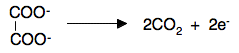So what you should now do is combine that with the manganate(VII) in acid half-equation to find the overall equation. If you have difficulties, look at the reaction between manganate(VII) in acid with hydrogen peroxide on the writing ionic equations for redox reactions page. You should find that the reacting proportions are 2MnO4- to 5(COO)22- ``` ``` Statement 28.2.9(b) This is for the common titration between acidified potassium manganate(VII) solution and a solution of an iron(II) salt. It would often be used to find the concentration of the iron(II) solution using potassium manganate(VII) solution which had been standardised using the reaction above. Practical details The potassium manganate(VII) solution is placed in the burette, and the solution containing the iron(II) salt is pipetted into a flask. The solution in the flask is acidified with some dilute sulfuric acid. The quantity isn't critical, but it has to be an excess. The purple potassium manganate(VII) solution is run in to the flask where it is initially decolourised. You continue until there is a very faint permanent pink colour. The equation You can find the half-equation for the manganate(VII) ions under acidic conditions either in the Data Book or on the page about writing ionic equations for redox reactions. The half-equation for the oxidation of Fe2+ to Fe3+ is trivial. Work out the overall equation now. You should find that the reacting proportions are 1MnO4- to 5Fe2+. Complicating things slightly You might also use this titration to estimate the amount of iron itself. Suppose you had some impure iron of a certain mass. If you dissolved this carefully in some dilute sulfuric acid, the iron will dissolve to give iron(II) ions in solution. You might then make this up to, say, 250 cm3 with pure water. If you then took a 25 cm3 sample of this and acidified it with more sulfuric acid, you could titrate it with standard potassium manganate(VII) solution, and use the results to find out how many moles of iron(II) ions you had in the original solution. That will be the same as the number of moles of iron in the impure iron. So you can work out the mass of the actual iron in your impure iron, and therefore the percentage purity of the iron. ``` ``` Statement 28.2.9(c) You will find all the details you need for this reaction on the page about copper chemistry. You only need the short section about half-way down titled "The reaction of hexaaquacopper(II) ions with iodide ions". ``` ``` Calculations These are all covered in detail in my calculations book, and all I can do now is give you a quick summary of how to do them. We will look again at the reaction between manganate(VII) ions and ethanedioate ions. Suppose the titration showed that 23.2 cm3 of potassium manganate(VII) solution was needed to react with 25.0 cm3 of sodium ethanedioate solution of concentration 0.0500 mol dm-3. Start with what you know most about With any titration calculation you start with what you know most about - in this case, the sodium ethanedioate solution. You know everything there is to know about it - its volume and its concentration. That means that you can work out how many moles of ethanedioate ions you have. Each mole of sodium ethanedioate has 1 mole of ethanedioate ions.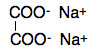If you had 1000 cm3 of the sodium ethanedioate, you would have 0.0500 moles of ethanedioate ions (that's the concentration you are given). So in 25.03 of solution you will have . . .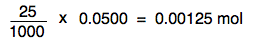Look at the reacting proportions The equation shows that 2MnO4- react with 5(COO)22- That means that the number of moles of manganate(VII) in the reaction will be 2/5 of the number of moles of ethanedioate ions. So the number of moles of manganate(VII) ions must be . . .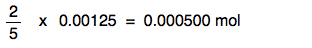Work out the concentration you want That number of moles of manganate(VII) ions was contained in the 23.2 cm3 that was added from the burette. All you need to do is to scale that up to find out how many moles there are in 1000 cm3 . . .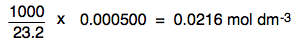That is to 3 significant figures. All the numbers you are working with are to that accuracy. ``` ``` Statement 28.2.10 This simply allows CIE to ask you about any similar example they might invent. The chemistry involved may not be familiar to you - but that doesn't matter. They will give you all the information you need. Don't panic! You need to be able to work out an overall equation from given half-equations, or half-equations that you can get from the Data Booklet. You need to be confident about doing straightforward titration calculations. You need to practise as many past paper questions as you can find. ``` ``` Go to the Section 28 Menu . . . To return to the list of learning outcomes in Section 28 Go to the CIE Main Menu . . . To return to the list of all the CIE sections Go to Chemguide Main Menu . . . This will take you to the main part of Chemguide. © Jim Clark 2020